# Voltage Multiplier

It is in actuality a modified capacitor filter circuit (rectifier circuit) which makes a DC output voltage that is two or more than two times the AC peak input. In this section, we can look into full-wave voltage doubler, half-wave voltage doubler, voltage tripler and finally quadrupler.

## Half Wave Voltage Doubler

The input wave form, circuit diagram and output waveform is shown in figure 1. Here, all through the positive half cycle, the forward biased D1 diode conducts and diode D2 will be in off condition. In this time, the capacitor (C1) charges to VSmax (peak 2o voltage). All through the negative half cycle, the forward biased D2 diode conducts and D1 diode will be in off condition. In this time C2 will start charging.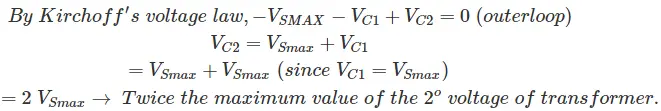Throughout the next positive half cycle, D2 is at reversed biased condition (open circuited). In this time C2 capacitor gets discharged through the load and thus voltage across this capacitor gets dropped.

But when there is no load across this capacitor, then both the capacitors will be at charged condition. That is C1 is charged to VSmax and C2 is charged to 2VSmax. Throughout the negative half cycle the C2 gets charged yet again (2VSmax). In the next half cycle, a half wave which is filtered by means of capacitor filter is obtained across the capacitor C2. Here, ripple frequency is same as the signal frequency. The DC output voltage of the order of 3kV can be obtained from this circuit.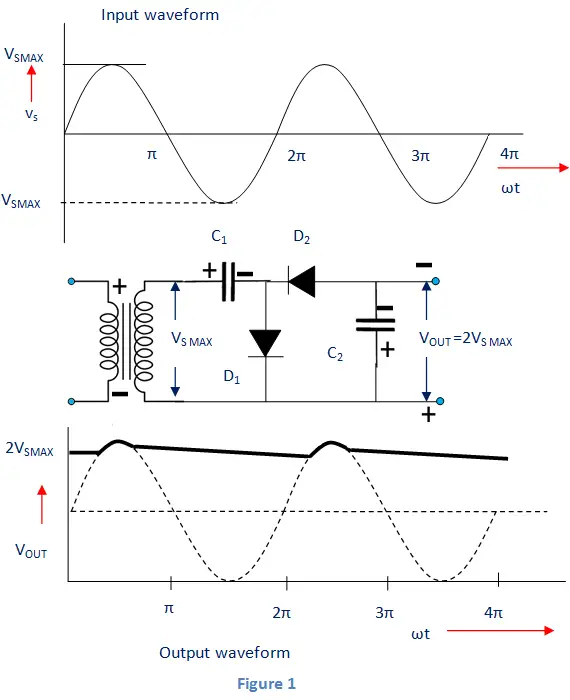## Full Wave Voltage Doubler

The input waveform of full-wave voltage doubler is shown below.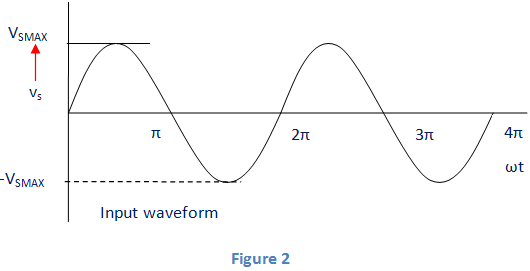The circuit diagram and output waveform is shown in figure 3. Here; all through the positive cycle of input voltage, the diode D1 will be in forward biased condition and capacitor C1 will gets charged to VSmax(peak voltage). At this time, D2 will be in reverse biased condition. All through the negative cycle of input voltage, the D2 diode will be in forward biased condition and the capacitor C2 gets charged. If the load is not connected across the output terminals, the total voltages of both the capacitors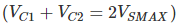are obtained as the output voltage. If some load is connected across the output terminals, then output voltage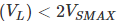.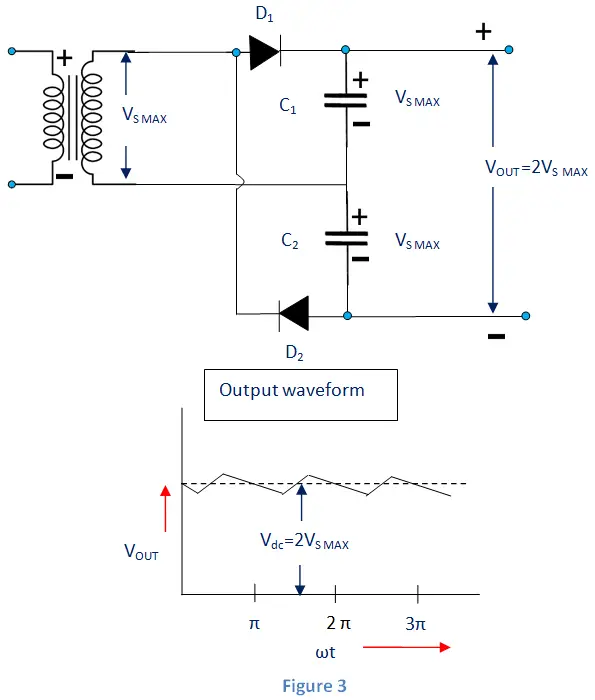We can see that, both the half-wave and full-wave voltage doubler will give 2VS MAX as output. It does not require any centre-tapped transformer. The peak inverse voltage rating of diodes will be equal to 2VS MAX. When compared to half wave voltage doubler, the full-wave voltage doubler can simply filter high frequency ripples and output ripple frequency will be equal to twice the supply frequency. But the problem in full-wave voltage doubler is that; in between the input and output, the common ground is absent.

Using the method of extension of half-wave voltage doubler circuit, any voltage multipliers (Tripler, Quadrupler etc) can be created. When both the capacitor leakage and load are small, we can achieve tremendously high DC voltages by means of these circuits that include several sections to step-up (increase) the DC voltage.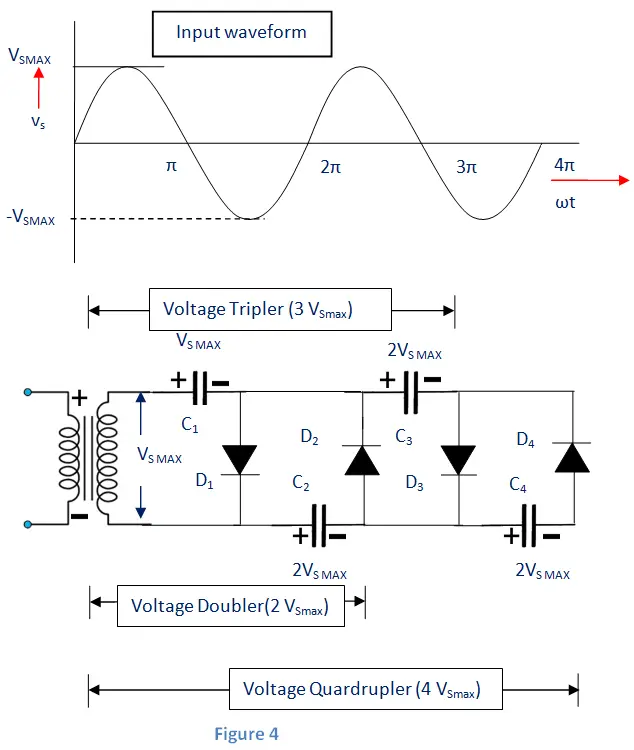Here; all through the first positive and negative half cycle is same as that of half-wave voltage doubler. Throughout the next positive half cycle, D1 and D3 conducts and C3 charges to 2VSmax. Throughout the next negative half cycle, D2 and D4 conducts and C4 charges to 2VSmax. When more diodes and capacitors are added, every capacitor will get charged to 2VSmax. At the output; odd multiples of VSmax can be attained, if measured from the top of transformer 2o winding and even multiples of VSmax can be attained, if measured from bottom of 2o winding of transformer.

### Application of Voltage Multiplier

• Cathode ray tubes.
• Cathode ray tubes in oscilloscope, Television receivers, Computer display.
• X-Ray systems
• Lasers
• Ion pumps
• Copy machines
• Electrostatic systems
• Photomultiplier tubes
• Travelling wave tubes (TWT)
• And several other devices which involves low current and high voltage applications.

Want To Learn Faster? 🎓
Get electrical articles delivered to your inbox every week.
No credit card required—it’s 100% free.